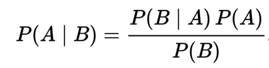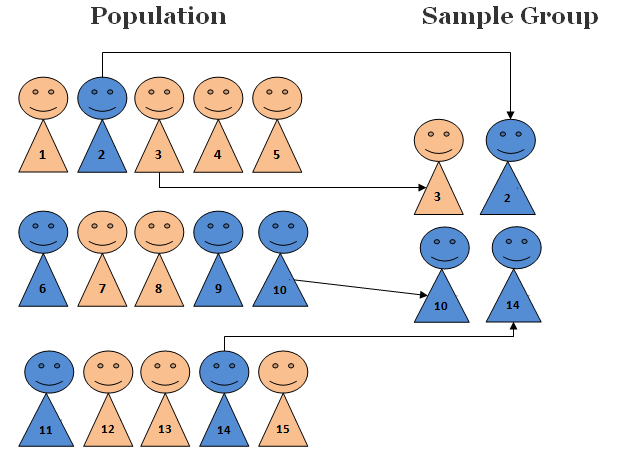# 3 Data Science Theorems every programmer should know

If you are aspiring towards data science career, three points below will bring a smile on your face, for sure:

a)  Harvard Business review has declared data scientist job as the sexiest job of the 21st century.

b) IBM predicts demand for data scientists will soar 28% by 2020.

c)  Glassdoor lists data scientist job as the #1 job in the U.S, with a median salary of around \$1,08,000, and a satisfaction rate of 4.3 out of 5.

Data science career potential undoubtedly is promising.

In the same context, I would like to ask an important question. What do you think, apart from programming (Python, R, or SAS), what skill will you need the most to become a data scientist?

Yes! You got it right, it’s math and statistics. (Don’t worry if you suck at math. There are absolutely creative tutorials available to upskill you in math).  Fundamentally, Mathematics is the base of all contemporary discipline of science and data science is not an exception too. Almost all the techniques of modern data science, including machine learning, carry some deep mathematical and statistical concepts as their supporting structure.

But, what does it mean when we say mathematics and statistics are essential skills to pursue a career in data science or AI? Should our youth, to prepare for a data-driven career, be spending their days deep in the fundamentals of a probability distribution, regression, and differential calculus?

No, it’s not like that but you need to have a basic understanding of underlying principles as well as statistics theorems, useful in creating data science and machine learning models.

In this blog post, we are going to discuss three data science theorems, in-depth with an example, every programmer should know to derive accurate result in an AI system.Alright. This is going to be super interesting and fun!

1.  Bayes Theorem: In front of the incredible powers of machine learning, we have become unfaithful to statistics, isn’t it true? But, can you imagine a career in the field of data science and ML, without prior knowledge of statistics especially probability theory.

image credit: https://www.analyticsvidhya.com

That is why I choose to discuss Bayes theorem in this blog post. So let’s get started.

Think of it and there is no chance that you never heard of this theorem before. Bayes’ theorem is the most important rules of probability theory hence also found its way in AI & machine learning, to form one of the highly decorated ML algorithms, named Naïve Bayes algorithm.

This theorem provides us the way to examine the probability of an event based on the prior knowledge of any event, related to the former one. Following equation gives the basic representation of the Bayes’ theorem, considering A and B are two related events:Image credit: Wikipedia

Here,

P(A|B): the conditional probability or the probability of event A to occur given that B occurred. This is also called the posterior probability.

P(B|A):  the probability of event B to occur given that A occurred.

P(A), P(B): the probability of event A or B to occur. It is also called prior probability.

Let’s take a simple example to get more insight into Bayes’ theorem.

Suppose you asked to pick a single card from a deck of playing cards. The probability that the card is a Jack is 4/52 as there are 4 jacks in a deck of 52 playing cards. In other words, we can say that the prior probability P(Jack) = 4/52 = 1/13

But, what if the evidence is provided, say someone looks at the card, that the picked card is a face card. In this case, the posterior probability i.e. P(Jack |Face) can be calculated using Bayes’ theorem:

P(Jack | Face) =  (P(Face | Jack))/(P(Face))  * P(Jack)

P(Face | Jack) will be 1 because every jack is also a face card.

P(Face | Jack), the probability of a face card will be 3/13 because there are three face cards(Jack, Queen, and King) in each suit.

From these values, the likelihood ratio i.e. (P(Face | Jack))/(P(Face)) will be 13/3.

Now, after putting all the values, Bayes’ theorem gives P(Jack | Face) = 1/3.

Still wondering how Bayes’ theorem suits well to the purpose of Machine Learning?  Well, let’s take the simplest ML model, where we need to make our model learn from a given set of attributes and then form a hypothesis to a response variable. Further, we use this hypothesis to predict a response, given a new set of a new instance. Here, Bayes’s theorem makes this possible for machine learning.

Moreover, if we talk about applications of Bayes’ Theorem then it is the base of spam filtering.

2.  Central Limit Theorem: Although Abraham de Moivre, a French-born mathematician, suggested central limit theorem (CLT) several centuries back, it continues to be applied to a great extent, especially in data science and machine learning algorithms.

Nuts & Bolts of Central Limit Theorem:

Before diving into its formal definition, let’s understand CLT and its working, with the help of an example.

Suppose, the total number of students in a school is 2500 and your task is to calculate the average height of all the students. How you can do this?

You might like

The most obvious approach we get from aspiring data scientist is to simply calculate the average:

a) First, measure the height of all, 2500 students.

c) Finally, divide the total sum of heights with the total number of students and all done, we will get the average.

Don’t you think measuring the height of all the students is going to be a very tiresome and long process? So, is there any alternate approach? Yes, let’s have a look:

a) First, draw the random group of students from school and call this a sample. Draw multiple samples, each consisting of 30 or more students.Image credit: https://research-methodology.net

b) Now, calculate the individual mean of all these samples.

c) Next, calculate the mean of these sample means.

d) The value we got here is the approximate mean height of the students in the school.

e) Graphically, the sample mean height of students will be a bell-shaped curve i.e. normal distribution.

To make a long story short, this is what the Central Limit Theorem is all about. Interesting? So, let’s go further and put a formal definition to CLT:

The Central Limit Theorem, a key concept in probability, states that with large sample size, the sampling distribution of the samples means approaches a normal distribution — no matter what the shape of the original population distribution.

It’ simply saying, as you take more samples, especially large ones, the graph of the sample means will take a bell-shaped curve i.e. to look like a normal distribution as shown below:

Image credit: https://www.thoughtco.com

Above fact holds especially true for the sample sizes over 30.

Mathematically, we can define CLT with the help of following formula:

µx=µ

And

σx=  σ/√n

Where,

µ = Population means

σ = Population standard deviation

µx = Sample mean

σx = Sample standard deviation

n = Sample size

The most important implication of CLT in machine learning is to inform the solution to linear algorithms such as linear regression.

3.  No-Free-Lunch (NFL) Theorem: Can we have a machine learning algorithm that works well with any kind of data? The answer is No, we can’t. The reason behind this is the theorem called No-Free-Lunch theorem.

It says, there is no one ML algorithm that works best for every problem. That is why in machine learning, we try multiple models and choose one that works best for a problem.

Unfortunately, there is no such thing like free lunch!Image Credit: https://www.thedailymeal.com

We can get more insight into no-free-lunch theorem with the help of following the simple example. Suppose we are asked to predict the next number from the sequence below:

A = 1,3,9,…

By assuming, the sequence at each time step is being generated by At = 3At-1,  most of us would probably predict 27 at the next place in the sequence.

On the other hand, there is no such reason to not believe the hypothesis that this sequence is simply the output of a random number generator. And if you think it as the other way, we cannot disapprove this hypothesis without seeing all the data points. Agreed?

That’s all for now, readers! Hope this blog post proves to be insightful for you! We would love to hear your thoughts too. Don’t hesitate to leave your comments in the section below. If you would like a career in Data Science, then our comprehensive course with live sessions, assessments, and placement assistant might be your best bet